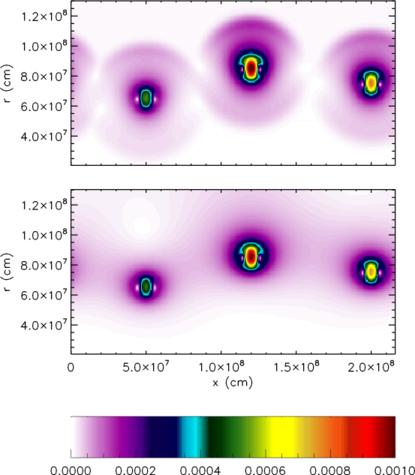|

## ∗ IntroductionFigure 1: Mach number for 3 burning rising bubbles from a compressible code (top) and a low Mach number hydrodynamics method (bottom).

A large number of interesting astrophysical phenomena occur at low Mach numbers. Evolving these flows with a fully compressible simulation code is inefficient, because of the need to follow the sound waves. For an explicit time-discretization (i.e., the new state is expressed solely in terms of the present state), a fundamental limitation exists on the size of the allowable timesteps -- the CFL condition. A timestep is restricted such that information may only propagate across one zone in the computational grid per timestep. In compressible flow, information propagates at the speeds: u, u + c, and u - c, where c is the sound speed. Mathematically the timestep restriction is expressed as

Δ t < min { Δ x / (|u| + c) }
For very low Mach number flows, this is
Δ t ~ Δ x / c
This means that for an interface moving at a Mach number M << 1, it takes 1/M timesteps for that interface to move just one zone!

Maestro solves a reformulation of the equations of hydrodynamics that filters out sound waves, while retaining the compressibility effects important to the problem at hand. This results in a timestep constraint of the form

Δ t < min{ Δ x / |u| }
Therefore, Maestro requires far fewer timesteps (~1/M fewer) to simulate low Mach number flows.

Mathematically, we can think of low Mach number flows as exhibiting instantaneous acoustic equilibriation. Figure 1 shows this graphically. Three temperature perturbations were placed in a stratified atmosphere, seeding nuclear reactions. The heat release makes the bubbles buoyant. The top panel shows the Mach number for the compressible solution—a clear signal resulting from the finite propagation speed of the soundwaves is seen surrounding each bubble. In the low panel, a low Mach number method was used. Owing to the instantaneous acoustic equilibriation, the velocity field is realized throughout the whole domain—even far from the bubbles. However, the flow at the bubbles is identical between the two codes, showing that in this case, the soundwaves are not imporatant to the dynamics.

Maestro was developed in a series of papers listed here.

Maestro is built on an adaptive mesh framework through the AMReX library and uses MPI and OpenMP for parallelization.

## ∗ Getting Started

Maestro is under active development.

To download the current version, visit the download page. For help, visit the help page.

There is an extensive User's Guide in the Maestro/Docs/ directory of the Maestro distribution to introduce the basics of the code.